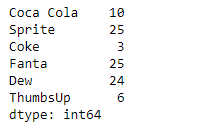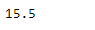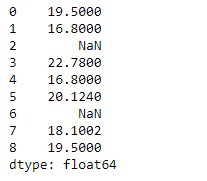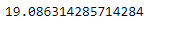Python | Pandas Series.mean()

• Last Updated : 11 Feb, 2019

Pandas series is a One-dimensional ndarray with axis labels. The labels need not be unique but must be a hashable type. The object supports both integer- and label-based indexing and provides a host of methods for performing operations involving the index.

Pandas Series.mean() function return the mean of the underlying data in the given Series object.

Syntax: Series.mean(axis=None, skipna=None, level=None, numeric_only=None, **kwargs)

Parameter :
axis : Axis for the function to be applied on.
skipna : Exclude NA/null values when computing the result.
level : If the axis is a MultiIndex (hierarchical), count along a particular level, collapsing into a scalar.
numeric_only : Include only float, int, boolean columns.
**kwargs : Additional keyword arguments to be passed to the function.

Returns : mean : scalar or Series (if level specified)

Example #1: Use Series.mean() function to find the mean of the underlying data in the given series object.

 # importing pandas as pdimport pandas as pd  # Creating the Seriessr = pd.Series([10, 25, 3, 25, 24, 6])  # Create the Indexindex_ = ['Coca Cola', 'Sprite', 'Coke', 'Fanta', 'Dew', 'ThumbsUp']  # set the indexsr.index = index_  # Print the seriesprint(sr)

Output :Now we will use Series.mean() function to find the mean of the given series object.

 # return the meanresult = sr.mean()  # Print the resultprint(result)

Output :As we can see in the output, the Series.mean() function has successfully returned the mean of the given series object.

Example #2: Use Series.mean() function to find the mean of the underlying data in the given series object. The given series object also contains some missing values.

 # importing pandas as pdimport pandas as pd  # Creating the Seriessr = pd.Series([19.5, 16.8, None, 22.78, 16.8, 20.124, None, 18.1002, 19.5])  # Print the seriesprint(sr)

Output :Now we will use Series.mean() function to find the mean of the given series object. we are going to skip all the missing values while calculating the mean.

 # return the mean# skip all the missing valuesresult = sr.mean(skipna = True)  # Print the resultprint(result)

Output :As we can see in the output, the Series.mean() function has successfully returned the mean of the given series object.

My Personal Notes arrow_drop_up